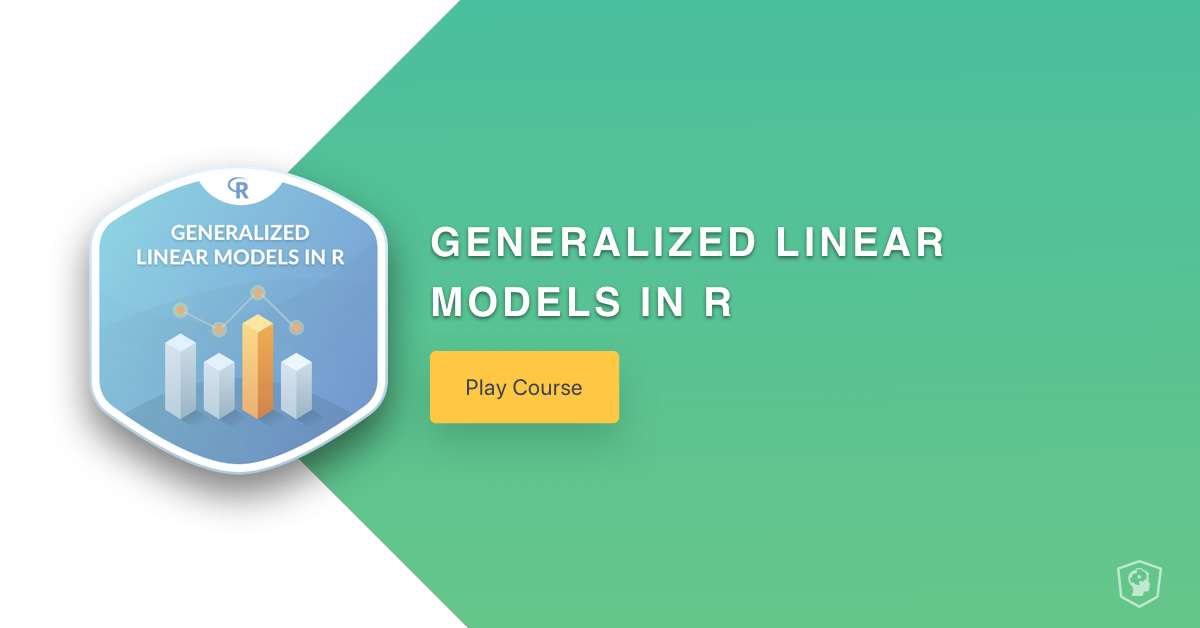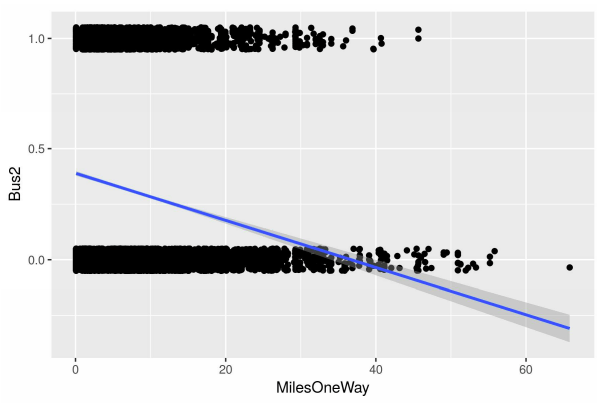# New Course: Generalized Linear Models in R

Learn about our new R course.
Sep 2018  · 2 min readHere is the course link.

## Course Description

Linear regression serves as a workhorse of statistics, but cannot handle some types of complex data. A generalized linear model (GLM) expands upon linear regression to include non-normal distributions including binomial and count data. Throughout this course, you will expand your data science toolkit to include GLMs in R. As part of learning about GLMs, you will learn how to fit model binomial data with logistic regression and count data with Poisson regression. You will also learn how to understand these results and plot them with ggplot2.

## Chapter 1: GLMs, an extension of your regression toolbox (Free)

This chapter teaches you how generalized linear models are an extension of other models in your data science toolbox. The chapter also uses Poisson regression to introduce generalize linear models.

## Chapter 2: Logistic Regression

This chapter covers running a logistic regression and examining the model outputs.## Chapter 3: Interpreting and visualizing GLMs

This chapter teaches you about interpreting GLM coefficients and plotting GLMs using ggplot2.

## Chapter 4: Multiple regression with GLMs

In this chapter, you will learn how to do multiple regression with GLMs in R.